### D.33 Quan­tum field de­riva­tions

This de­riva­tion will find the prop­er­ties of a sys­tem de­scribed by a Hamil­ton­ian of the form: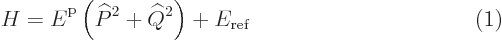Hereand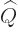are Her­mit­ian op­er­a­tors with com­mu­ta­tor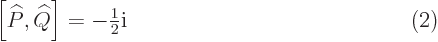andand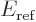are con­stants with units of en­ergy.

First note that the com­mu­ta­tor (2) di­rectly im­plies the un­cer­tainty re­la­tion­ship, chap­ter 4.5.2 (4.46):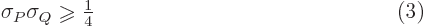Also note that the evo­lu­tion equa­tions for the ex­pec­ta­tion val­ues ofand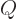fol­low di­rectly from chap­ter 7.2 (7.4). The com­mu­ta­tor ap­pear­ing in it is read­ily worked out us­ing the com­mu­ta­tor (2) and the rules of chap­ter 4.5.4. Since en­ergy eigen­states are sta­tion­ary, ac­cord­ing to the evo­lu­tion equa­tions in such states the ex­pec­ta­tion val­ues ofandwill have to be zero.

The equal­ity of theandterms in the Hamil­ton­ian is a sim­ple mat­ter of sym­me­try. Noth­ing changes if you swapand, adding a mi­nus sign for one. Then un­avoid­ably the two terms in the Hamil­ton­ian must be equal; it is shown be­low that the eigen­func­tions are unique.

The com­mu­ta­tor (2) also im­plies that,, and all their com­bi­na­tions, do not com­mute with the Hamil­ton­ian. So they are not con­served quan­ti­ties of the sys­tem. How­ever, there are two com­bi­na­tions,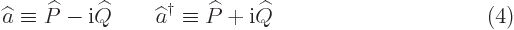whose com­mu­ta­tor with the Hamil­ton­ian gives back a mul­ti­ple of the same thing:In other words,andare com­mu­ta­tor eigen­op­er­a­tors of the Hamil­ton­ian. The above re­la­tions are read­ily checked us­ing the given com­mu­ta­tor (2) and the rules of chap­ter 4.5.4.

To see why that is im­por­tant, mul­ti­ply both sides of the eigen­value prob­lems above with a sys­tem en­ergy eigen­func­tion of en­ergy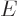:Af­ter writ­ing out the de­f­i­n­i­tions of the com­mu­ta­tors, rec­og­niz­ing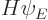as, and re­ar­rang­ing, that gives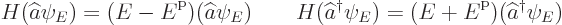These re­sults can be com­pared to the de­f­i­n­i­tion of an en­ergy eigen­func­tion. Then it is seen thatis an en­ergy eigen­func­tion with one unitless en­ergy than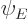. And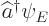is an en­ergy eigen­func­tion with one unitmore en­ergy than. So ap­par­entlyandact as an­ni­hi­la­tion and cre­ation op­er­a­tors of quanta of en­ergy. They act as shown to the left in fig­ure A.6.

There are how­ever two im­por­tant caveats for these state­ments. Iforis zero, it is not an en­ergy eigen­func­tion. Eigen­func­tions must be nonzero. Also, even if the statesorare not zero, they will not nor­mally be nor­mal­ized states.

To get a bet­ter un­der­stand­ing of these is­sues, it is help­ful to first find the Hamil­ton­ian in terms ofand. There are two equiv­a­lent forms,These ex­pres­sions can be ver­i­fied by plug­ging in the de­f­i­n­i­tions (4) ofandand us­ing the com­mu­ta­tor (2). (Note that sub­tract­ing the two ex­pres­sions gives the com­mu­ta­tor ofandto be 1.)

Now look at the first Hamil­ton­ian first. Ifwould be zero for some state, then that state would have en­ergy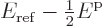. But that is not pos­si­ble. If you look at the orig­i­nal Hamil­ton­ian (1), the en­ergy must at least be; square Her­mit­ian op­er­a­tors are non­neg­a­tive.

(To be more pre­cise, if you square a Her­mit­ian op­er­a­tor, you square the eigen­val­ues, mak­ing them non­neg­a­tive. It is said that the square op­er­a­tor is pos­i­tive def­i­nite, or, if there are zero eigen­val­ues, pos­i­tive semi-def­i­nite. And such an op­er­a­tor pro­duces non­neg­a­tive ex­pec­ta­tion val­ues. And the ex­pec­ta­tion val­ues of the op­er­a­tors in the Hamil­ton­ian do add up to the to­tal en­ergy; just take an in­ner prod­uct of the Hamil­ton­ian eigen­value prob­lem with the wave func­tion. See chap­ter 4.4 for more in­for­ma­tion on ex­pec­ta­tion val­ues.)

It fol­lows thatis never zero. This op­er­a­tor can be ap­plied in­def­i­nitely to find states of higher and higher en­ergy. So there is no max­i­mum en­ergy.

But there is a pos­si­bil­ity thatis zero. As the sec­ond form of the Hamil­ton­ian in (5) shows, that re­quires that the en­ergy of stateequals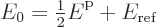Now if you start from any en­ergy stateand ap­plysuf­fi­ciently many times, you must even­tu­ally end up at this en­ergy level. If not, you could go on low­er­ing the en­ergy for­ever. That would be in­con­sis­tent with the fact that the en­ergy can­not be lower than. It fol­lows that the above en­ergy is the low­est en­ergy that a state can have. So it is the ground state en­ergy.

And any other en­ergy must be a whole mul­ti­ple ofhigher than the ground state en­ergy. Oth­er­wise you could not end up at the ground state en­ergy by ap­ply­ing. There­fore, the en­ergy eigen­states can be de­noted more mean­ing­fully by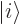rather than. Here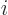is the num­ber of quantathat the en­ergy is above the ground state level.

Now as­sume that the ground state is unique. In that case, there is one unique en­ergy eigen­func­tion at each en­ergy level. That is a con­se­quence of the fact that if you go down a unit in en­ergy withand then up a unit again with, (or vice versa), you must end up not just at the same en­ergy, but at the same state. Oth­er­wise the state would not be an eigen­func­tion of the Hamil­ton­ian in one of the forms given in (5). Re­peated ap­pli­ca­tion shows that if you go down any num­ber of steps, and then up the same num­ber of steps, you end up at the same state. Since every state must end up at the unique ground state, every state must be the re­sult of ap­ply­ingto the ground state suf­fi­ciently many times. There is just one such state for each en­ergy level.

If there are two in­de­pen­dent ground states, ap­ply­ingon each gives two sep­a­rate sets of en­ergy eigen­states. And sim­i­lar if there are still more ground states. Ad­di­tional sym­bols will need to be added to the kets to keep the dif­fer­ent fam­i­lies apart.

It was al­ready men­tioned that the states pro­duced by the op­er­a­torsandare usu­ally not nor­mal­ized. For ex­am­ple, the state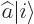will have a square mag­ni­tude given by the in­ner prod­uct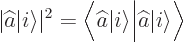Now if you takeorto the other side of an in­ner prod­uct, it will change into the other one; the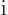in the de­f­i­n­i­tions (4) will change sign. So the square mag­ni­tude ofbe­comes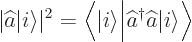From the sec­ond form of the Hamil­ton­ian in (5), it is seen that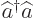gives the num­ber of en­ergy quanta. And since the stateis nor­mal­ized, the square mag­ni­tude ofis there­fore. That means thatwhere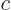is some num­ber of mag­ni­tude 1. Sim­i­larly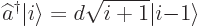But note that you can al­ways change the de­f­i­n­i­tion of an en­ergy eigen­func­tion by a con­stant of mag­ni­tude 1. That al­lows you, while go­ing up from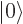us­ing, to re­de­fine each state so that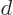is 1. And ifis al­ways one, then so is. Oth­er­wisewould not be.

In the ground state, the ex­pec­ta­tion val­ues ofandare zero, while the ex­pec­ta­tion val­ues of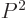and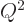are equal to the min­i­mumal­lowed by the un­cer­tainty re­la­tion (3). The de­riva­tions of these state­ments are the same as those for the har­monic os­cil­la­tor ground state in {D.13}.